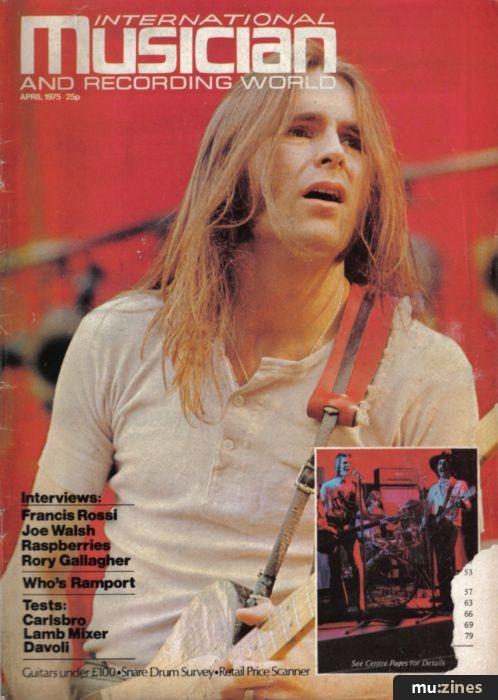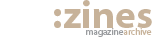•## Discussing Decibels

The answer to 'how much length?' is in centimetres, inches, feet or miles. The answer to 'how much weight?' is in pounds, grammes, tons or tonnes. But which unit should you use to answer 'how much bass control?' 'how much amplifier gain?' or 'how much change in sound level?' We are now dealing with the decibel.

No one has to use decibels. Anything which can be expressed in decibels can also be expressed in other ways. If we want to say 'How much treble control?' for example, we can start by choosing a pure tone in the treble range; decide how much power output we would get for a given input if the control had not been fitted at all; then with the treble control fitted and the knob fully one way we could find the note is, say, ten times more powerful and turned fully the other way, only one tenth as powerful. This is from 10 to 1 up to 1 to 10 down; giving a range of 100 to 1. You will note that to get the range we multiplied the ratio up by the ratio down.

It so happens that a power ratio of 10 to 1 could also be expressed as 10 dB (10 decibels) so we can say that the control will give from 10dB up to 10 dB down, or from an increase of 10 dB to a decrease of 10 dB, more neatly, +10 dB to —10 dB. The + meaning up and the — meaning down. We now find the first big difference between using decibels and using ratios. The control range is the difference between +10 dB and —10 dB which is 20 dB which, of course means exactly the same as the power ratio of 100:1 we got above. Where you would multiply or divide the power ratios you add or subtract the decibel values.

The second difference is; when the control is set to neither increase nor decrease the power level compared with not having a control at all, we could say we have multiplied the signal by one; or; that we are half-way between +10 dB and —10 dB which is 0 dB.

Times one is 0dB. 0dB means no change in power level; 10dB means 10 times the power; 20dB means 100 times the power; 30 dB means 1000 times the power etc. In the other direction —10dB is one tenth of the power; —20dB is 1/100th pf the power; —30dB is 1/1000ths of the power and so on.

The difference between +60 dB and —20 dB is 80 dB or the difference between one million times the power and one hundredth of the power is one hundred million to one. 80dB is equivalent to 100,000,000 to 1 power ratio!! The third difference is very big numbers or very small numbers can be expressed without using lots of '0's.

If I say I am going to change the sound level by 13dB; we know that 10 dB is a power ratio of 10 and 20 dB is a power ratio of 100 so 13dB must be somewhere in between. To explain just where and also to demonstrate some very interesting properties of decibels we are now going to look at them from a totally different point of view.

Suppose we sat in a room with an amplifier and a signal source. We set the output to one watt and then to two watts and we agree to call this change 'one loudness step'. If I then ask you to increase the volume 'one loudness step' at a time and secretly noted the power level at each step, we would get a relationship between amplifier power and loudness steps like that shown in figure 1. Steps 7, 8, 9 and 10 would be at 128 watts, 256W, 512W and 1024W. We could also go down in loudness steps to a very low level before giving up because it is "too quiet to hear". So although the first steps are only 1 and 2 watts we need go only 10 steps to require over 1000 watts. Similarly, if we went down in volume, we would only go down 10 steps to be at less than 1/1000ths of a watt. Notice the similarity with the decibel scale?

The only difference in fact, is the size of the steps chosen. Each step is twice the height of the last one and half the size of the next one for no reason other than we chose the first steps to be one and two watts. We could have chosen any other power levels we liked.

Another point to notice about figure 1 is that if we change the scale by rubbing out all the numbers; put step 0 in another position, say where step 4 is at present; then change the power scale to suit; all the other steps still have the correct relative size. In other words, if we magnify any part of the curve drawn through the centres of the steps, in a vertical direction, it will exactly fit the same curve at same point further up. If we magnify the curve by a thousand times in both directions, the bottom thousandth of the new curve would exactly fit the whole of the curve we started with!

Now we are going to keep the curve but rub out the loudness steps and replace them with another set of smaller size which will be decibel steps. For convenience let us set 0dB at one watt again. I emphasise that we can set 0dB at any reference level we like. 10dB will be 10 times 1 watt, or 10 watts, and 20 dB will be 100 times 1 watt or 100 watts. If we put these two columns in their places and evenly fill the gaps between with the right number of columns we then have a gap of dB steps. (figure 2)

Obviously we can't draw many steps because they rapidly become so small we could not see them or so big they wou|d spill over the edge of the paper (and jam the printing machine). The answer is to change the way we draw the graph so the power scale shrinks as the power gets bigger. If we get the amount of shrinking correct, the curved line through the steps can be made to look straight. (figure 3)

On changing the graph around I would have changed the words 'Output Power in Watts' to 'Power Ratio' because any number of watts can be chosen as the starting point. Decibels only say how big one power level is compared with another. They don't define how much power unless you say what power level you are calling 0dB. What reference levels to use will be discussed when we come to examples of dBs in action.

Figure 4 shows the intermediate steps between 0dB and 10dB in terms of power ratio. If you want something bigger than 10dB you just add another '0' for every extra 10dB you have. For example; 63dB is the same as 60dB plus 3dB. Look up 3dBs in figure 4; this is a power ratio of 2. Now add six noughts because 60dB is a power ratio of 1,000,000.

Therefore 63dB is a power ratio of 2,000,000. It's easy, isn't it? Read through the artic|e once more then resolve not to ever be confused by decibels again.

Next month I shall be explaining decibe|s in action.

Battersea Rock

###### Next article in this issue

Guitars Under £100

#### International Musician & Recording World - Copyright: Cover Publications Ltd, Northern & Shell Ltd.International Musician - Apr 1975

Donated & scanned by: Mike Gorman

Should be left alone:

Topic:

#### Sound Fundamentals

Feature by Bruce Gibbs

Previous article in this issue:

Next article in this issue:

##### Help Support The Things You Love

mu:zines is the result of thousands of hours of effort, and will require many thousands more going forward to reach our goals of getting all this content online.

If you value this resource, you can support this project - it really helps!

#### If you're enjoying the site, please consider supporting me to help build this archive...

##### ...with a one time Donation, or a recurring Donation of just £2 a month. It really helps - thank you!